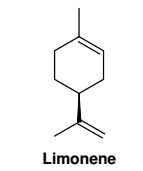# Problem: What is the  unsaturation number of limonene? A. 0 B. 1 C. 2 D. 3 E. 4 F. 5 G. 6 H. 7 I. 8 J. 9

🤓 Based on our data, we think this question is relevant for Professor Choudhury's class at BC.

###### Problem Details

What is the  unsaturation number of limonene?

A. 0

B. 1

C. 2

D. 3

E. 4

F. 5

G. 6

H. 7

I. 8

J. 9What scientific concept do you need to know in order to solve this problem?

Our tutors have indicated that to solve this problem you will need to apply the Degrees of Unsaturation concept. You can view video lessons to learn Degrees of Unsaturation. Or if you need more Degrees of Unsaturation practice, you can also practice Degrees of Unsaturation practice problems.

What is the difficulty of this problem?

Our tutors rated the difficulty ofWhat is the  unsaturation number of limonene? A. 0 B. 1 C...as low difficulty.

How long does this problem take to solve?

Our expert Organic tutor, Jonathan took 1 minute and 32 seconds to solve this problem. You can follow their steps in the video explanation above.

What professor is this problem relevant for?

Based on our data, we think this problem is relevant for Professor Choudhury's class at BC.